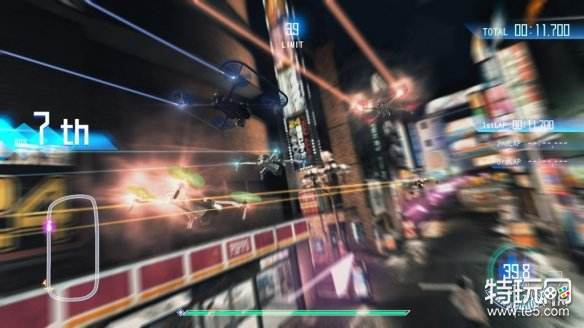# 《审判之眼死神的遗言》作家第二个谜题答案是什么？作家第二个谜题答案一览

111×2=3

11×99=4

222×0=1

3×4=2

111×3=?

12×99=?

333×0=?

5×6=?